Search by Topic

Resources tagged with Summation of series similar to Integral Sandwich:

Filter by: Content type:
Age range:
Challenge level:

There are 30 results

Broad Topics > Patterns, Sequences and Structure > Summation of seriesSummats Clear

Age 16 to 18 Challenge Level:

Find the sum, f(n), of the first n terms of the sequence: 0, 1, 1, 2, 2, 3, 3........p, p, p +1, p + 1,..... Prove that f(a + b) - f(a - b) = ab.2^n -n Numbers

Age 16 to 18

Yatir from Israel wrote this article on numbers that can be written as $2^n-n$ where n is a positive integer.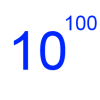Googol

Age 16 to 18 Short Challenge Level:

Find the smallest value for which a particular sequence is greater than a googol.Proof Sorter - Geometric Series

Age 16 to 18 Challenge Level:

Can you correctly order the steps in the proof of the formula for the sum of a geometric series?More Polynomial Equations

Age 16 to 18 Challenge Level:

Find relationships between the polynomials a, b and c which are polynomials in n giving the sums of the first n natural numbers, squares and cubes respectively.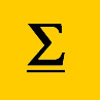The Kth Sum of N Numbers

Age 16 to 18

Yatir from Israel describes his method for summing a series of triangle numbers.Picture Story

Age 14 to 16 Challenge Level:

Can you see how this picture illustrates the formula for the sum of the first six cube numbers?OK! Now Prove It

Age 16 to 18 Challenge Level:

Make a conjecture about the sum of the squares of the odd positive integers. Can you prove it?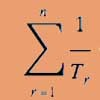Reciprocal Triangles

Age 16 to 18 Challenge Level:

Prove that the sum of the reciprocals of the first n triangular numbers gets closer and closer to 2 as n grows.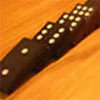An Introduction to Mathematical Induction

Age 16 to 18

This article gives an introduction to mathematical induction, a powerful method of mathematical proof.Sum the Series

Age 16 to 18

This article by Alex Goodwin, age 18 of Madras College, St Andrews describes how to find the sum of 1 + 22 + 333 + 4444 + ... to n terms.The Root of the Problem

Age 14 to 18 Challenge Level:

Find the sum of this series of surds.Slick Summing

Age 14 to 16 Challenge Level:

Watch the video to see how Charlie works out the sum. Can you adapt his method?Speedy Summations

Age 16 to 18 Challenge Level:

Watch the video to see how to add together an arithmetic sequence of numbers efficiently.Harmonically

Age 16 to 18 Challenge Level:

Is it true that a large integer m can be taken such that: 1 + 1/2 + 1/3 + ... +1/m > 100 ?Vanishing Point

Age 14 to 18 Challenge Level:

How can visual patterns be used to prove sums of series?Clickety Click and All the Sixes

Age 16 to 18 Challenge Level:

What is the sum of: 6 + 66 + 666 + 6666 ............+ 666666666...6 where there are n sixes in the last term?Telescoping Series

Age 16 to 18 Challenge Level:

Find $S_r = 1^r + 2^r + 3^r + ... + n^r$ where r is any fixed positive integer in terms of $S_1, S_2, ... S_{r-1}$.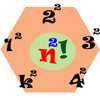Seriesly

Age 16 to 18 Challenge Level:

Prove that k.k! = (k+1)! - k! and sum the series 1.1! + 2.2! + 3.3! +...+n.n!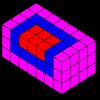Summing Squares

Age 14 to 16 Challenge Level:

Discover a way to sum square numbers by building cuboids from small cubes. Can you picture how the sequence will grow?Overarch 2

Age 16 to 18 Challenge Level:

Bricks are 20cm long and 10cm high. How high could an arch be built without mortar on a flat horizontal surface, to overhang by 1 metre? How big an overhang is it possible to make like this?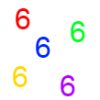Clickety Click

Age 16 to 18 Short Challenge Level:

What is the sum of: 6 + 66 + 666 + 6666 ............+ 666666666...6 where there are n sixes in the last term?Seriesly

Age 16 to 18 Short Challenge Level:

Prove that k.k! = (k+1)! - k! and sum the series 1.1! + 2.2! + 3.3! +...+n.n!A Swiss Sum

Age 16 to 18 Challenge Level:

Can you use the given image to say something about the sum of an infinite series?Double Trouble

Age 14 to 16 Challenge Level:

Simple additions can lead to intriguing results...Summing Geometric Progressions

Age 14 to 18 Challenge Level:

Watch the video to see how to sum the sequence. Can you adapt the method to sum other sequences?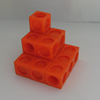Sums of Powers - A Festive Story

Age 14 to 18

A story for students about adding powers of integers - with a festive twist.Degree Ceremony

Age 16 to 18 Challenge Level:

What does Pythagoras' Theorem tell you about these angles: 90°, (45+x)° and (45-x)° in a triangle?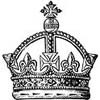Succession in Randomia

Age 16 to 18 Challenge Level:

By tossing a coin one of three princes is chosen to be the next King of Randomia. Does each prince have an equal chance of taking the throne?Production Equation

Age 16 to 18 Challenge Level:

Each week a company produces X units and sells p per cent of its stock. How should the company plan its warehouse space?